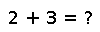# fldmod

fldmod(x, y)

The floored quotient and modulus after division. Equivalent to `(fld(x,y), mod(x,y))`.

## Examples

``````julia> fldmod(5, 2)
(2,1)

julia> fldmod(5, -2)
(-3,-1)

julia> fldmod(-5, 2)
(-3,1)``````

Checking you are not a robot: